Courses

# Dimensions (Formulae and Equations) - Units and Measurements, Class 11, Physics | EduRev Notes

## NEET : Dimensions (Formulae and Equations) - Units and Measurements, Class 11, Physics | EduRev Notes

The document Dimensions (Formulae and Equations) - Units and Measurements, Class 11, Physics | EduRev Notes is a part of the NEET Course Physics Class 11.
All you need of NEET at this link: NEET

DIMENSIONAL FORMULAE AND DIMENSIONAL ANALYSIS
In describing physical things and events quantitatively, we refer to numerical values of base quantities, and also introduce numbers derived by inserting these values into certain mathematical formulas.

Definition: Dimensions of a physical quantity are the power to which the fundamental quantities must be raised to represent the given physical quantity.
For example, density  = (mass/volume) = mass/(lenght)3
or
density = (mass) (length)-3  ...(i)
Thus, the dimensions of density are 1 in mass and -3 in length. The dimensions of all other fundamental quantities are zero.
For convenience, the fundamental quantities are represented by one letter symbols. Generally mass is denoted by M, length by L, time by T and electric current by A.

The thermodynamic temperature, the amount of substance and the luminous intensity are denoted by the symbols of their units K, mol and cd respectively.
The physical quantity that is expressed in terms of the base quantities is enclosed in square brackets.
[sinθ] = [cosθ] = [tanθ] = [ex] = [M0L0T0]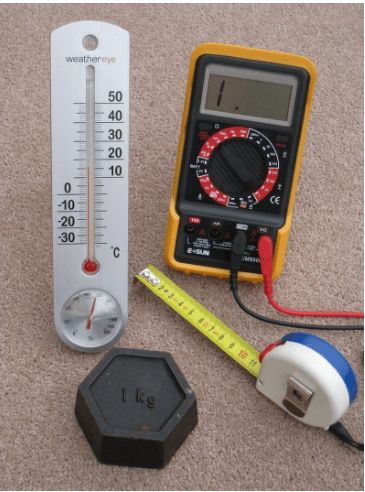Fig: Four metric measuring devices: a tape measure in centimetres, a thermometer in degrees Celsius, a kilogram weight and a multimeter that measures volts, amperes and ohms

DIMENSIONAL FORMULA
It is an expression which shows how and which of the fundamental units are required to represent the unit of physical quantity.
For example:
By definition, Velocity is a derived quantity. We can write its dimensional formula using:
Velocity = Displacement/time
Now, displacement is measured in Length, [L1] and time is measured in [T1] which are both fundamental quantities. Hence, we can represent the dimensional formula of velocity as:
[v] = [s/t] = [L/T] = [LT-1]
Similarly, we can evaluate dimensional formula of all physical derived quantities. The table given below shows some of the most occurring physical quantities and their dimensions.

Different quantities with units. symbol and dimensional formula.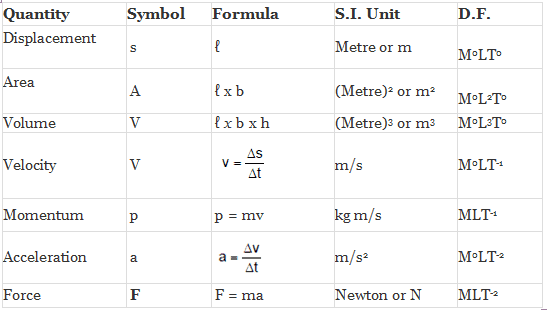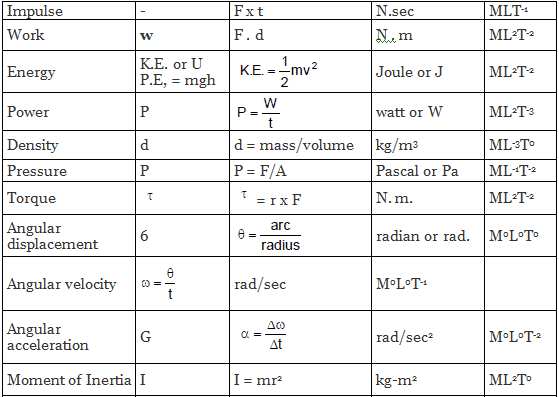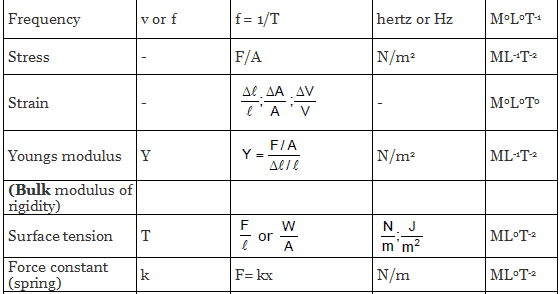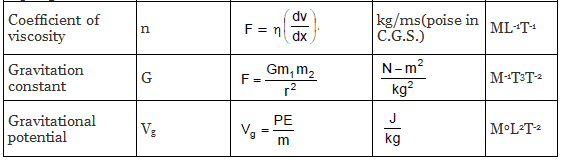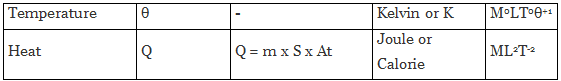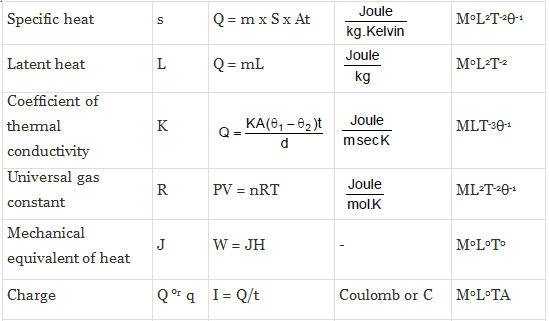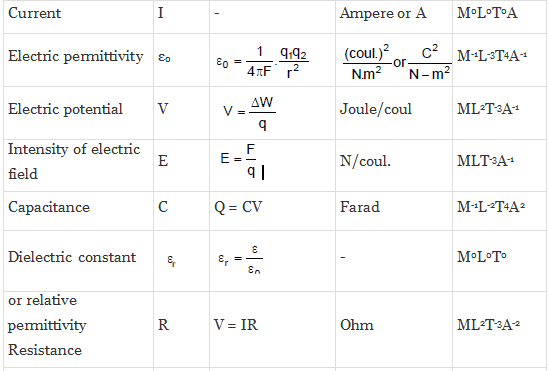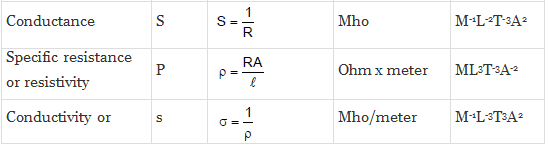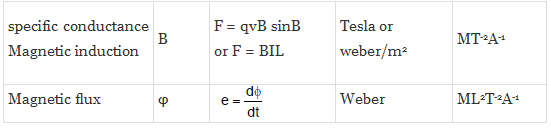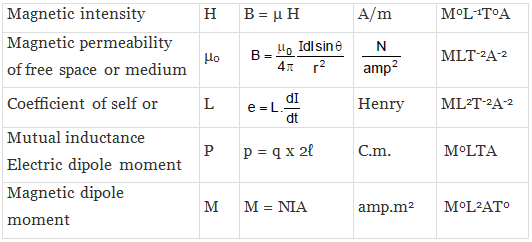Applications of Dimensional Analysis
(a) Conversion of units:
This is based on the fact that the product of the numerical value (n) and its corresponding unit (u) is a constant, i.e.,
n[u] = constant
or n1[u1] = n2 [u2]
Suppose the dimensions of a physical quantity are a in mass, b in length and c in time. If the fundamental units in one system are M1, L1 and T1 and in the other system are M2, L2 and T2 respectively. Then we can write.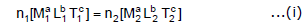Here n1 and n2 are the numerical values in two system of units respectively. Using Eq. (i), we can convert the numerical value of a physical quantity from one system of units into the other system.

Example 1.  The value of gravitation constant is G = 6.67 × 10-11 Nm2/kg2 in SI units. Convert it into CGS system of units.
Solution. The dimensional formula of G is [M-1 L3 T-2].
Using equation number (i), i.e.,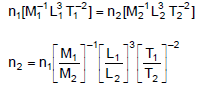Here, n1 = 6.67 x 10-11
M1 = 1 kg, M2 = 1 g = 10-3 kg, L1  = 1 m, L2 = 1 cm = 10-2 m, T1 = T2 = 1s
Substituting in the above equation, we get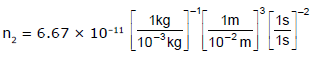or n2 = 6.67 x 10-8
Thus, value of G in CGS system of units is 6.67 x 10-8 dyne cm2/g2.

(b) To check the dimensional correctness of a given physical equation:
Every physical equation should be dimensionally balanced. This is called the 'Principle of Homogeneity'. The dimensions of each term on both sides of an equation must be the same. On this basis we can judge whether a given equation is correct or not. But a dimensionally correct equation may or may not be physically correct.

Example 2. Show that the expression of the time period T of a simple pendulum of length l given by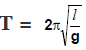is dimensionally correct.
Solution.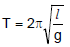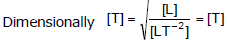As in the above equation, the dimensions of both sides are same. The given formula is dimensionally correct.

(c) Principle of Homogeneity of Dimensions.
This principle states that the dimensions of all the terms in a physical expression should be same. For example, in the physical expression s = ut + 1/2 at2,  the dimensions of s, ut and 1/2 at2 all are same.

Note: The physical quantities separated by the symbols +, -, =, >, < etc., have the same dimensions.

Example 3. The velocity v of a particle depends upon the time t according to the equation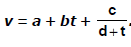Write the dimensions of a, b, c and d.
Solution.
From principle of homogeneity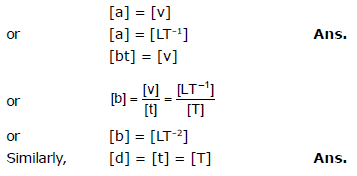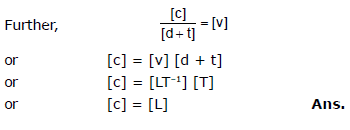(d) To establish the relation among various physical quantities:
If we know the factors on which a given physical quantity may depend, we can find a formula relating the quantity with those factors. Let us take an example.

Example.4. The frequency (f) of a stretched string depends upon the tension F (dimensions of force), length l of the string and the mass per unit length m of string. Derive the formula for frequency.
Solution. Suppose, that the frequency f depends on the tension raised to the power a, length raised to the power b and mass per unit length raised to the power c. Then.
f ∝ [F]a[l]b[μ]c
or f = k[F]a[l]b[μ]c
Here, k is a dimensionless constant. Thus,
[f] = [F]0[l]b[μ]c
or [M0L0T-1] = [MLT-2]a[L]b[ML-1]c
or [M0L0T-1] = [Ma+c La+b-c T-2a]
For dimensional balance, the dimension on both sides should be same.
Thus,    a + c = 0    ...(ii)
a + b - c = 0    ...(iii)
and    - 2a = - 1    ...(iv)
Solving these three equations, we get
a = 1/2, c = (-1/2) and b = -1
Substituting these values in Eq. (i), we get
f = k(F)1/2(l)-1(μ)-1/2
or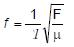Experimentally, the value of k is found to be 1/2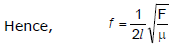Limitations of Dimensional Analysis
The method of dimensions has the following limitations:
(a) By this method the value of dimensionless constant can not be calculated.
(b) By this method the equation containing trigonometrical, exponential and logarithmic terms cannot be analysed.
(c) If a physical quantity depends on more than three factors, then relation among them cannot be established because we can have only three equations by equalizing the powers of M, L and T.

Offer running on EduRev: Apply code STAYHOME200 to get INR 200 off on our premium plan EduRev Infinity!

## Physics Class 11

75 videos|223 docs|152 tests

,

,

,

,

,

,

,

,

,

,

,

,

,

,

,

,

,

,

,

,

,

,

,

,

,

,

,

;